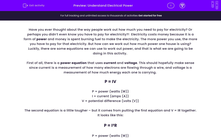# Understand Electrical Power

In this worksheet, students will learn the meaning of the term power and apply this to electrical circuit problems using the power equation.Key stage:  KS 4

Year:  GCSE

GCSE Boards:   AQA, AQA Trilogy, AQA Synergy, OCR 21st Century, OCR Gateway, Pearson Edexcel, Eduqas,

Popular topics:   Physics worksheets

Difficulty level:#### Worksheet Overview

Have you ever thought about the way people work out how much you need to pay for electricity? Or perhaps you didn’t even know you have to pay for electricity?!  Electricity costs money because it is a form of power and money is spent burning fuel to make the electricity. The more power you use, the more you have to pay for that electricity. But how can we work out how much power one house is using? Luckily, there are some equations we can use to work out power, and that is what we are going to be doing in this activity.

First of all, there is a power equation that uses current and voltage. This should hopefully make sense since current is a measurement of how many electrons are flowing through a wire, and voltage is a measurement of how much energy each one is carrying.

P = IV

P = power (watts (W))
I = current (amps (A))
V = potential difference (volts (V))

The second equation is a little tougher – but it comes from putting the first equation and V = IR together. It looks like this:

P = I²R

P = power (watts (W))
I = current (amps (A))
R = resistance (ohms (Ω))

Let’s take a look at an example:

Question: A kettle uses 400 V with a current of 3 A. Calculate the power of the kettle.

Step 1   Highlight all of the numbers in the equation:

A kettle uses 400 V with a current of 3 A. Calculate the power of the kettle.

Step 2   Write out the numbers next to their symbols:

P = ?
I = 3 A
V = 400 V

Step 3   Put the numbers into the equation:

P = IV
P = 3 x 400

P = 1,200 W

Let’s try some questions on this!

### What is EdPlace?

We're your National Curriculum aligned online education content provider helping each child succeed in English, maths and science from year 1 to GCSE. With an EdPlace account you’ll be able to track and measure progress, helping each child achieve their best. We build confidence and attainment by personalising each child’s learning at a level that suits them.

Get started